## Ward Leonard Drive Transfer Function:

In the classical Ward Leonard Drive Transfer Function, the dc drive motor having constant excitation is supplied from a variable voltage generator, as shown in Fig. 6.9. The generator is driven at constant speed. The field current of the dc generator is varied by varying the field voltage to have variable voltage at the terminals, which is ultimately fed to the motor. A transfer function relating the speed of the motor and generator field voltage is required.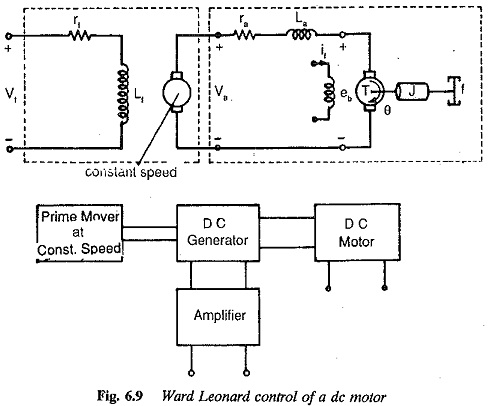The voltage induced in the armature of the generator is given by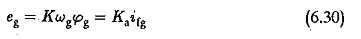where Ka is a constant which includes the constancy of generator speed and the proportionality constant between field current and air gap flux. As usual the effects of saturation are neglected. Eliminating ifg from Eq. 6.30 we have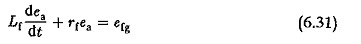The transfer function between the armature voltage and field voltage of the generator is given by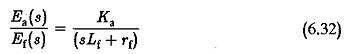The transfer function of the armature controlled motor is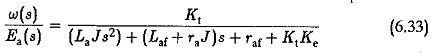Combining Eqs 6.32 and 6.33, we have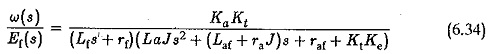Neglecting the effects of La the transfer function simplifies in the second order to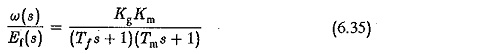where

K= (Ka/rf) generator gain constant

T= Lf/rf generator field time constant

Km=Kt/(raf+KtKe) motor gain constant

T= raJ/(raf+KtKe) motor time constant

The block diagrams of a generator and a motor are shown in Fig. 6.10.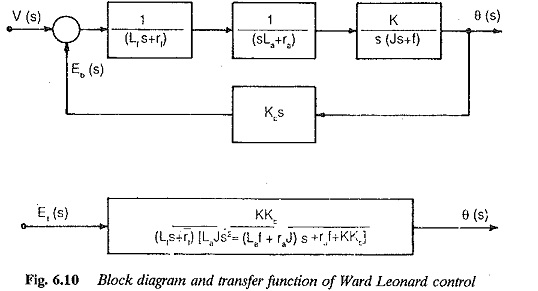Alternately: The equations of the system may be derived in the state vari­able form taking if,ia and ω as the state variables. A signal flow graph may be drawn to represent these equations. Using Mason’s gain formula the transfer function may be derived.

Using the schematic of Fig. 6.9 representing a Ward Leonard speed control system we have for the generator field circuit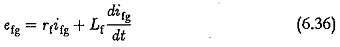which can be rearranged as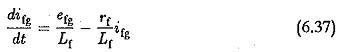For the loop of armatures the circuit equation is given by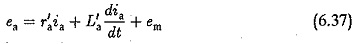ea is the voltage induced in the armature of the generator and is given by Kgifg.

r′a and L′a include resistance and inductance of both generator and motor armatures respectively.

em is the back emf of the motor given by Kmω. Substituting in Eq. (6.37) and rearranging the terms we have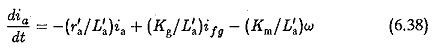The torque balance equation gives the dynamics of the motor. It is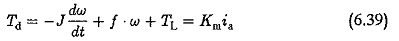Rearranging the terms we have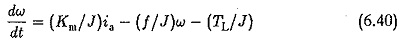Equations 6.40 are the state equations of the system and given in matrix ro­tation as

Scroll to Top